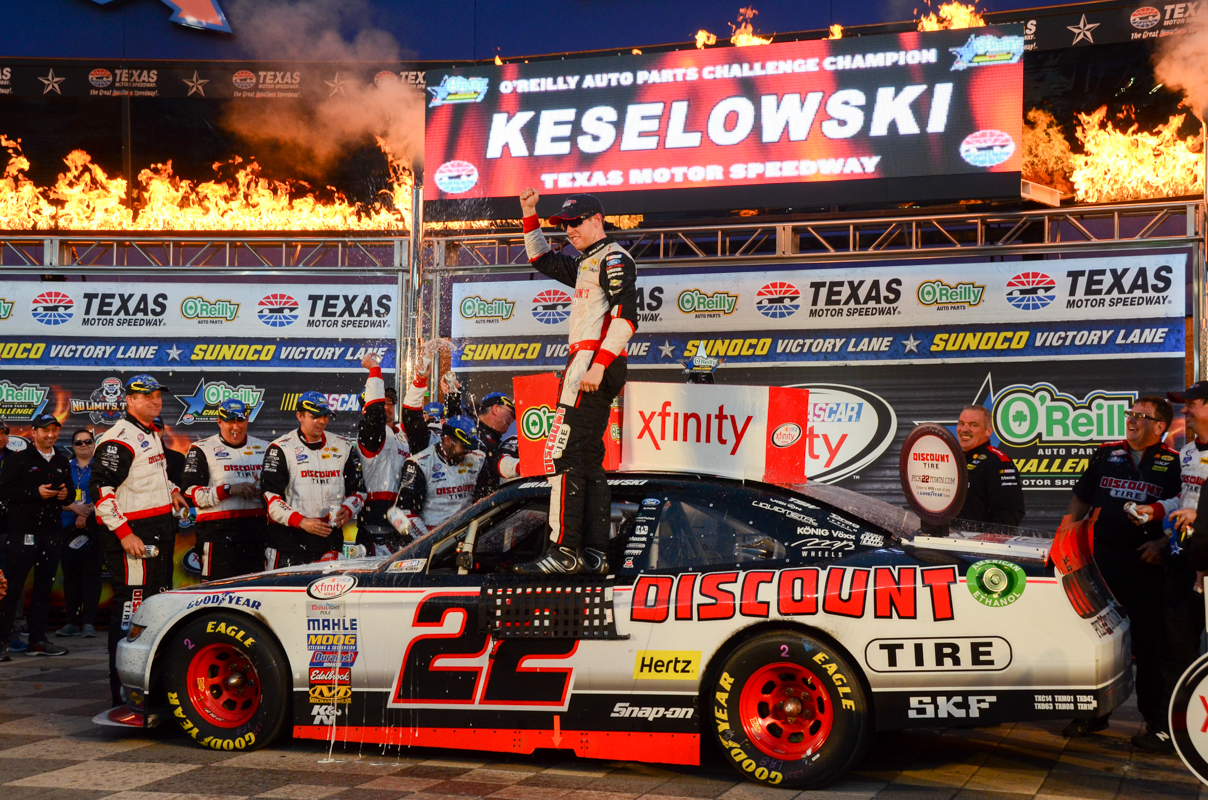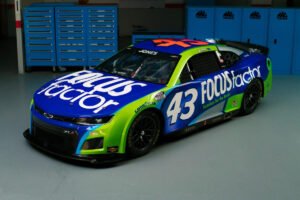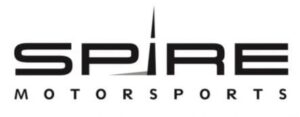November 29, 2021

# OnPitRoad.com Pick ‘Em Fantasy Pick Results for O’Reilly Auto Parts ChallengeThe Picks

Ronald Costigan and Ashley McCubbin picked Chase Elliott, Kyle Busch and Kevin Harvick. Elliott finished eighth with Busch 14th and Harvick second. Points: 36+31+43=110

Doug Townsend and Justin Tucker picked Brad Keselowski, Kyle Busch and Chris Buescher. Keselowski won with Buescher 11th. Points: 48+31+33=112

Robert Hill picked Darrell Wallace Jr., Erik Jones and Ryan Sieg. Wallace finished 19th with Jones fourth and Sieg 13th. Points: 25+40+32=97

Tim Johnston, Marshall Gabell and Brooke Clarke picked Chase Elliott, Brad Keselowski and Kevin Harvick. Points: 36+48+43=127

Kreistina Blinova picked Ty Dillon, Austin Dillon and Kevin Harvick. Ty finished fifth with Austin third. Points: 39+42+43=124

Kyle Magda picked Derek White, Brad Keselowski and Daniel Suarez. Suarez finished sixth with White 31st. Points: 13+48+38=99

Jeffrey Breazeale and Steve Love picked Regan Smith, Brad Keselowski and Kyle Busch. Smith finished ninth. Points: 35+48+31=114

Jeni Harris picked Darrell Wallace Jr., Regan Smith and Chase Elliott. Points: 25+35+36=96

Brandon Feeney picked Ty Dillon, Kyle Busch and Kevin Harvick. Points: 39+31+43=113

Parker Ledger picked Regan Smith, Chase Elliott and Kevin Harvick. Points: 35+36+43=114

Rory Townsend picked Chase Elliott, TJ Bell and Kevin Harvick. Bell finished 38th. Points: 36+6+43=85

Alex George picked Chase Elliott, Kyle Larson and Kevin Harvick. Larson finished 33rd. Points: 36+12+43=91

Mike Cousins picked Ty Dillon, Chris Buescher and Kevin Harvick. Points: 39+33=43=115

Stephen Placido picked Darrell Wallace Jr., Brad Keselowski and Kevin Harvick. Points: 25+48+43=106

The Results

1. Tim Johnston, Marshall Gabell and Brooke Clarke – 127
2. Kreistina Blinova – 124
3. Mike Cousins – 115
4. Jeffrey Breazeale, Parker Ledger and Steve Love – 114
5. Brandon Feeney – 113
6. Doug Townsend and Justin Tucker – 112
7. Ronald Costigan and Ashley McCubbin – 110
8. Stephen Placido – 106
9. Kyle Magda – 99
10. Robert Hill – 97
11. Jeni Harris – 96
12. Alex George – 91
13. Rory Townsend – 85

Current Fall XFINITY Series Standings

1. (Points Leader Post Chicago) Steve Love – 590+114=704
2. Justin Tucker – 578+112=690 (-14)
3. Jeffrey Breazeale – 570+114=684 (-20)
4. Brandon Feeney – 563+113=676 (-28)
5. Ronald Costigan – 563+110=673 (-31)
6. Doug Townsend – 555+112=667 (-37)
7. Jeni Harris – 560+96=656 (-48)
8. Ashley McCubbin – 543+110=653 (-51)
9. Parker Ledger – 519+114=633 (-71)
10. Kreistina Blinova – 508+124=632 (-72)
11. Robert Hill – 529+97=626 (-78)
12. Tim Johnston, Marshall Gabell and Brooke Clarke – 497+127=624 (-80)
13. Mike Cousins – 500+115=615 (-89)
14. Stephen Placido – 498+106=604 (-100)
15. Alex George – 465+91=556
16. Kyle Magda – 418+99=517
17. Rory Townsend – 379+85=464

Full Season XFINITY Series Standings

2. Ronald Costigan – 3419+110=3529 (-57)
3. Jeffrey Breazaele – 3377+114=3491 (-95)
4. Ashley McCubbin – 3360+110=3470
5. Brandon Feeney – 3325+113=3438
6. Doug Townsend – 3299+112=3411
7. Steve Love – 3289+114=3403
8. Brooke Clarke – 3169+127=3296
9. Tim Johnston – 3161+127=3288
10. Stephen Placido – 3172+106=3278
11. Kyle Magda – 3125+99=3224
12. Mike Cousins – 3076+115=3191
13. Parker Ledger – 3073+114=3177
14. Marshall Gabell – 3039+127=3166
15. Kreistina Blinova – 3041+124=3165
16. Jeni Harris – 3127+96=3123
17. Robert Hill – 3009+97=3106
18. Alex George – 2869+91=2960
19. Rory Townsend – 2702+85=2787

Fall Overall Standings

1. (Points Leader Post Cup Martinsville) Steve Love – 1655+82+114=1851
2. Doug Townsend – 1636+79+112=1827 (-24)
3. Brandon Feeney – 1587+86+113=1786 (-65)
4. Justin Tucker – 1577+89+112=1778 (-73)
5. Jeni Harris – 1580+86+96=1762 (-89)
6. Parker Ledger – 1543+90+114=1747
7. Brooke Clarke – 1536+82+127=1745
8. Ronald Costigan – 1522+76+110=1708
9. Ashley McCubbin – 1506+89+110=1705
10. Kreistina Blinova – 1470+82+124=1676
11. Tim Johnston – 1481+66+127=1674
12. Mike Cousins – 1466+81+115=1662
13. Stephen Placido – 1467+82+106=1655
14. Marshall Gabell – 1432+64+127=1623
15. Jeffrey Breazeale – 1433+68+114=1615
16. Alex George – 1395+78+91=1564
17. Rory Townsend – 1400+69+85=1554
18. Kyle Magda – 1247+89+99=1435
19. Robert Hill – 1216+74+97=1387

Full Season Overall Points Standings

1. (Points Leader Post Cup Kansas) Justin Tucker – 8343+89+112=8544
2. Ronald Costigan – 8153+76+110=8339 (-205)
3. Ashley McCubbin – 8070+89+110=8269
4. Brandon Feeney – 8070+86+113=8269
5. Doug Townsend – 8046+79+112=8237
6. Steve Love – 7991+82+114=8187
7. Stephen Placido – 7899+82+106=8088
8. Parker Ledger – 7830+90+114=8034
9. Brooke Clarke – 7788+82+127=7997
10. Tim Johnston – 7754+66+127=7947
11. Mike Cousins – 7691+81+115=7887
12. Jeffrey Breazeale – 7685+68+114=7867
13. Kreistina Blinova – 7618+82+124=7834
14. Kyle Magda – 7571+89+99=7759
15. Marshall Gabell – 7567+64+127=7758
16. Jeni Harris – 7583+86+96=7715
17. Alex George – 7495+78+91=7664
18. Rory Townsend – 7247+69+85=7401
19. Robert Hill – 6738+74+97=6909

#### You may have missed#### Why Progressive Insurance is the right choice#### FOCUSFACTOR® TO PARTNER WITH RICHARD PETTY MOTORSPORTS#### Spire Motorsports to Field NASCAR Camping World Truck Series Team in 2022